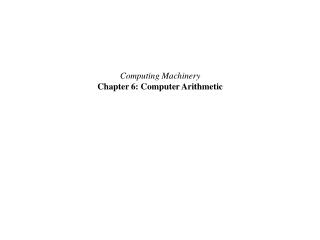Download PresentationComputing Machinery Chapter 6: Computer ArithmeticComputing Machinery Chapter 6: Computer Arithmetic - PowerPoint PPT Presentation

Download PresentationComputing Machinery Chapter 6: Computer Arithmetic
An Image/Link below is provided (as is) to download presentation

Download Policy: Content on the Website is provided to you AS IS for your information and personal use and may not be sold / licensed / shared on other websites without getting consent from its author. While downloading, if for some reason you are not able to download a presentation, the publisher may have deleted the file from their server.

- - - - - - - - - - - - - - - - - - - - - - - - - - - E N D - - - - - - - - - - - - - - - - - - - - - - - - - - -
Presentation Transcript

1. Computing Machinery Chapter 6: Computer Arithmetic

2. Integer Representations 0000000000000000000000000000000 = +0 0000000000000000000000000000001 = +1 0000000000000000000000000000010 = +2 : 0111111111111111111111111111111 = +2,147,483,647 1000000000000000000000000000000 = -0 1000000000000000000000000000001 = -1 1000000000000000000000000000010 = -2 1111111111111111111111111111111 = -2,147,483,647

3. Magnitudes of Binary Encoded Base Positions

4. Two's Complement 1. Generate the magnitude of the value in binary 2. Invert each bit of the binary number (0 becomes 1 and 1 becomes 0), called 1's complement 3. Add one (1) to the one's complement to produce the two's complement. (Ignore any overflow.) Example: -34 in two's complement 34/2 = 17 remainder 0 _ _ _ _ _ _ _ 0 17/2 = 8 remainder 1 _ _ _ _ _ _ 1 0 8/2 = 4 remainder 0 _ _ _ _ _ 0 1 0 4/2 = 2 remainder 0 _ _ _ _ 0 0 1 0 2/2 = 1 remainder 0 _ _ _ 0 0 0 1 0 1/2 = 0 remainder 1 _ _ 1 0 0 0 1 0 0/2 = 0 remainder 0 _ 0 1 0 0 0 1 0 0/2 = 0 remainder 0 0 0 1 0 0 0 1 0 0 0 1 0 0 0 1 0 <- magnitude of -34 1 1 0 1 1 1 0 1 <- one's complement + 1 1 1 0 1 1 1 1 0 <- two's complement

5. Converting Between Binary, Octal, and Hexadecimal binary octal hexadecimal decimal 0000 0 0 0 0001 1 1 1 0010 2 2 2 0011 3 3 3 0100 4 4 4 0101 5 5 5 0110 6 6 6 0111 7 7 7 1000 10 8 8 1001 11 9 9 1010 12 A 10 1011 13 B 11 1100 14 C 12 1101 15 D 13 1110 16 E 14 1111 17 F 15 01001100100111011111 01 001 100 100 111 011 111 1 1 4 4 7 3 7 0100 1100 1001 1101 1111 4 C 9 D F

6. Integer Addition and Subtraction

7. Finite Represenation in Two's Complement

8. IEEE Single-Precision Floating Point

9. IEEE Representation of p

10. IEEE Special Values

11. Integer Multiplication (Unsigned)

12. Integer Multiplication Hardware

13. Integer Multiplication (signed) Booth's Recoding Booth's recoding reduces the number of computations, which reduces the amount of hardware and time required to perform a multiplication standard

14. Booth's Multiplication 3 0011 M -M = 1101 x7 0111 Q A Q Q-1 N 0 0 0 0 0 1 1 1 0 4 +1 1 0 1 1 1 0 1 0 1 1 1 0 1 1 1 0 1 0 1 1 1 3 1 1 1 1 0 1 0 1 1 2 1 1 1 1 1 0 1 0 1 1 +0 0 1 1 0 0 1 0 1 0 1 0 1 0 0 0 1 0 1 0 1 0 0 00010101 = 21

15. Integer Division with remainder rebuilding the dividend, Q recurrence relation for bitwise integer division

16. Integer Division (restoring)

17. Integer Division (non-restoring) reformulating the recurrence relation restoring test can be done once at the end of the division

18. Booth's Integer Division

19. IEEE Floating-Point Multiplication

20. POWER2 Floating-Point Unit (FPU) Architecture The IBM POWER2 Floating-Point Unit is a hardware implementation of arithmetic operations on IEEE format floating-point numbers. "The FPU receives two instructions from the instruction cache unit (ICU). These two instructions go through a predecode stage where the FPU discards non-floating-point instructions. The MAF unit performs all of the floating-point arithmetic instructions, such as the multiply-add fused operation, as well as all floating-point store operations." http://www-03.ibm.com/servers/eserver/pseries/hardware/whitepapers/power/fpu.html

21. FPU Arithmetic Unit http://www-03.ibm.com/servers/eserver/pseries/hardware/whitepapers/power/fpu.html